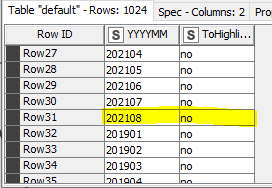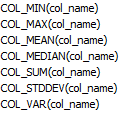# Column Expression add YES NO column highest value

Hi everyone I am using the Column Expression node to add a YES/NO column to identify the highest value in a column.

I am using two expressions (attempts) :

``````if(max(toInt(column("YYYYMM"))) == toInt(column("YYYYMM"))){
"yes"
}
else{
"no"
}
``````

and

``````if(colMax(toInt(column("YYYYMM"))) == toInt(column("YYYYMM"))){
"yes"
}
else{
"no"
}
``````

But, I am unable to get the proper result

Thank youYes No Col Max Column Expressions.knwf (22.0 KB)

Neither the `max` nor `colMax` functions in the Column Expressions node is able to process entire columns. You can test this yourself by evaluating the expression max(\$yourcolumnnamehere) or colMax(\$yorucolumnnamehere\$). Or you could read the function description.

One approach to solving this problem is to convert the strings to numbers, then use a Math Formula node to calculate the max column value, then either a Column Expressions or Rule Engine node to compare the values, then delete the unnecessary columns.

Another approach is to sort the strings in descending order, keep only the first row, convert it to a flow variable, then use a Column Expressions node to do the comparison

If your data has actual dates, then it might be worth exploring the KNIME Date&Time format as another option.

1 Like

Thank you, I guess that the Column Expression node must consider these Math functions@ScottF

Thank you

Hi @mauuuuu5 , most probably the reason why the Column Expressions node does not have these Math functions is because it can’t guarantee that the columns are numeric, since it has access to all columns of the input node, as opposed to the Math Formula node that pre-filters numeric columns only.

Just adding to your issue there, like any other nodes, the Column Expressions reads row-wise, that is horizontally, meaning each column of the same row. Of course, Knime will eventually process all the rows of the input node, but each row will only see or have access to the values in the columns of its row. Only the Python script allows you to navigate through a table.

Of course, as you found out with @elsamuel 's help, exceptionally the Math Formula node can give you the min, max, mean, etc (the functions you mentioned) of a column as long as the column is numeric.

So, the max() or colMax() functions from the Column Expression would be comparing values for the same row, that is why you could not achieve what you wanted to. And as @elsamuel suggested, you can check the description of the functions to understand how they work, and you can always just evaluate what both function returns.

Hi @bruno29a thank you for your response and clarification. I just wanted to make “everything” using a single node, but it does not matter if more nodes are needed.

Cheers

1 Like

Hello,

agree with @mauuuuu5, single node solution for this would be nice. I could only came up with 2 node solution. Use Rank node which will give you 1 where maximum is and follow it with Rule Engine to add yes/no.

Br,
Ivan

1 Like

This topic was automatically closed 7 days after the last reply. New replies are no longer allowed.